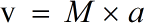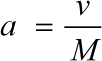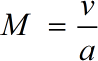Request a Tool

Mach Number Calculator

Mach Number is commonly used to represent the speed of an object when it is traveling close to or above the speed of sound.

Object Speed
0

Formula• v = Object Speed
• a = Speed of Sound
• M = Mach Number

Defination / Uses

The Mach Number is a dimensionalless value calculated as a ratio of an object's speed to the speed of sound in the surrounding environment. The symbol 'M' represents the Mach number.

Sound's Speed
0

Formula• a = Speed of Sound
• v = Object Speed
• M = Mach Number

Defination / Uses

The Mach Number is a dimensionalless value calculated as a ratio of an object's speed to the speed of sound in the surrounding environment. The symbol 'M' represents the Mach number.

Mach Number
0

Formula• M = Mach Number
• a = Speed of Sound
• v = Object Speed

Defination / Uses

The Mach Number is a dimensionalless value calculated as a ratio of an object's speed to the speed of sound in the surrounding environment. The symbol 'M' represents the Mach number.

How to use mach number calculator?

There are only two basic steps.

• First select the option from dropdown. What you need to calculate.
• Then add the other values in their corresponding fields.

Thats it! You will get your answer in less than a second. Use upper given formula in case of manual calculation.

The Mach number comes after the unit, so instead of "2 Mach" or Machs, the second Mach number is "Mach 2." When an item is going close to or over the speed of sound, the Mach Number is widely employed to indicate its speed. The Mach number is used to describe high-speed fluid flows inside channels such as nozzles, diffusers, and wind tunnels, as well as objects travelling at high speeds in a fluid. Sound travels at various rates in various mediums and at various temperatures. For quick calculation use our weetools. No sign-up, registration OR captcha is required to use this tool.